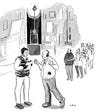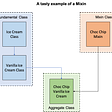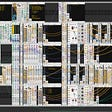# Numbers fly like bananas!

C# is a strongly typed language. Every expression that evaluates to a value belongs to a specific set, a particular type.

There is a set of built-in numeric types that are the bricks for creating new custom types. These new types are complex structures that retain behavior and qualities. So a typical C# program uses build-in types and user-defined ones.

In object-oriented programming, objects are instances of a specific “class”, and also custom types. Furthermore, it has a strong focus on behavior more than data, using polymorphism, either using “duck-typing” or interfaces.

Functional programming uses types as data. They do not have any specific behavior.

So what is a numeric type?

If we borrow the concept from Category Theory, a numeric type is a Monoid.

A monoid is a category with just one object. For instance, the type Int32 (at least in the .net framework) represents signed integers with values that range from negative 2,147,483,648 through positive 2,147,483,647, so a subset of the set of integers Z.

As opposed to set theory, category theory focuses not on elements but on points called objects and the relations between these objects called morphisms or arrows. It’s interesting to note that in this case, a natural number that could be regarded as an object is a morphism, while it exists only one object, the whole set of int32.

In F# we can use a sequence to represent a whole set of “infinite” numbers. A sequence is a logical series of elements all of one kind (type). Sequences are computed only when required. Hence a better performance when not all the elements are needed.

Sequences, in F#, and you should not be surprised to know that they are also a type, are represented by the seq<’T> type.

`module Monoid =     /// the identity arrow    let identity = 0    /// the set of int32    let arrows =  seq { -2147483647.. 2147483647 }    /// lookup for an arrow    let search number seq =  Seq.exists (fun s->s=number)seq      /// composition law        let ( >> ) A B = A + B`

This module satisfies all the characteristics of a category:

The Data:

1. a class of objects,
2. a collection of morphisms between pairs of objects;
3. a composition rule.

The Properties

1. Each object has an identity morphism.
2. The composition is associative
`module Test =    open Monoid    /// an example with the number 4    let number = -2147483647    printfn "Is the %A number an arrow? %A" number (search number arrows)     /// is the identity satisfied?    printfn "Is the number %A >> with identity %A equal to the number itself %A" number  identity (number >> identity)        /// associative law    let A = 1    let B = -23432    let C = 22    printfn "A=%A, B=%A, C=%A // (A >> B) >> C = A >> (B >> C) %A >> %A = %A >> %A // %A=%A"  A B C (A>>B) C A (B>>C)  ((A>>B)>>C) (A>>(B>>C))`

And finally the output:

Is the -2147483647 number an arrow? true

Is the number -2147483647 >> with identity 0 equal to the number itself -2147483647

A=1, B=-23432, C=22 // (A >> B) >> C = A >> (B >> C) -23431 >> 22 = 1 >> -23410 // -23409=-23409

Bananas are like boomerangs that don’t come back. Also, integers are morphisms that do not objectify themselves. We have been using F# and functional programming to show that a numeric type is a monoidal category.

--

--

--

## More from Mauro Ghiani

A complete non sequitur being.

Love podcasts or audiobooks? Learn on the go with our new app.

## Top 4 Cloud Adoption Trends That Will Shape Enterprise## How to streamline interactions between multiple repositories with multi-project pipelines## Kubernetes pod security 101## I'm going into Second Year CompSci and I have no clue what to do, Help!## Third Person: Unity learn## 2021: Taking the Swing## Hello world in Django## Mauro Ghiani

A complete non sequitur being.

## Mixins in Common Lisp## El Correo Libre Issue 46## Modifying riscv-xv6